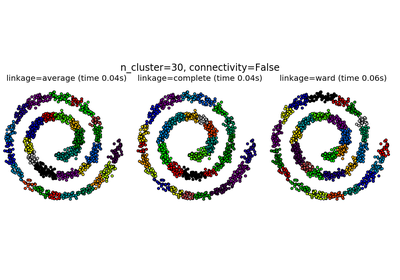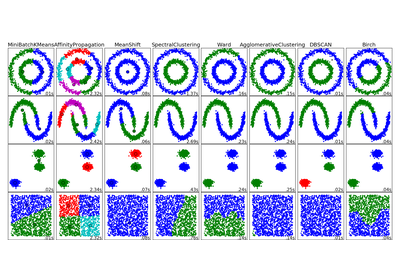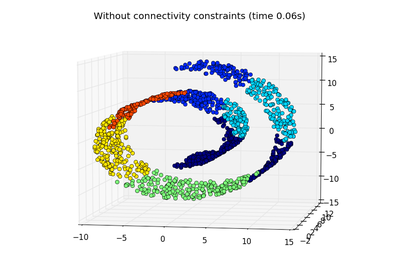# `sklearn.neighbors`.kneighbors_graph¶

`sklearn.neighbors.``kneighbors_graph`(X, n_neighbors, mode='connectivity', metric='minkowski', p=2, metric_params=None, include_self=False, n_jobs=1)[source]

Computes the (weighted) graph of k-Neighbors for points in X

Read more in the User Guide.

Parameters: X : array-like or BallTree, shape = [n_samples, n_features] Sample data, in the form of a numpy array or a precomputed `BallTree`. n_neighbors : int Number of neighbors for each sample. mode : {‘connectivity’, ‘distance’}, optional Type of returned matrix: ‘connectivity’ will return the connectivity matrix with ones and zeros, and ‘distance’ will return the distances between neighbors according to the given metric. metric : string, default ‘minkowski’ The distance metric used to calculate the k-Neighbors for each sample point. The DistanceMetric class gives a list of available metrics. The default distance is ‘euclidean’ (‘minkowski’ metric with the p param equal to 2.) include_self: bool, default=False. : Whether or not to mark each sample as the first nearest neighbor to itself. If None, then True is used for mode=’connectivity’ and False for mode=’distance’ as this will preserve backwards compatibilty. p : int, default 2 Power parameter for the Minkowski metric. When p = 1, this is equivalent to using manhattan_distance (l1), and euclidean_distance (l2) for p = 2. For arbitrary p, minkowski_distance (l_p) is used. metric_params: dict, optional : additional keyword arguments for the metric function. n_jobs : int, optional (default = 1) The number of parallel jobs to run for neighbors search. If `-1`, then the number of jobs is set to the number of CPU cores. A : sparse matrix in CSR format, shape = [n_samples, n_samples] A[i, j] is assigned the weight of edge that connects i to j.

Examples

```>>> X = [, , ]
>>> from sklearn.neighbors import kneighbors_graph
>>> A = kneighbors_graph(X, 2, mode='connectivity', include_self=True)
>>> A.toarray()
array([[ 1.,  0.,  1.],
[ 0.,  1.,  1.],
[ 1.,  0.,  1.]])
```

## Examples using `sklearn.neighbors.kneighbors_graph`¶Agglomerative clustering with and without structureComparing different clustering algorithms on toy datasetsHierarchical clustering: structured vs unstructured ward